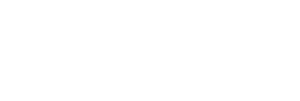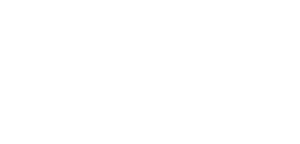Drag

# 经济代写｜ECON 210: Quantitative Methods for Economists Practice Final Exam

1. Suppose a firm with cost function C(q) = 9q2 faces demand curve p(q) = 1000 − q.

(a) What is its (total) revenue function, R(q)?
(b) What is its profit function, π(q)?
(c) Graph the profit function. Make sure all intercepts and axes are labeled.
(d) At what quantity is profit maximized?
(e) What is the optimal price?
(f) What is the maximized profit?
(g) Indicate this maximized profit on your diagram above.

2. Suppose f(x; y) = x2 − xy + y2 − 90x.

(a) What are the values of x and y at the turning point? Hint: There is no constraint for
this problem so you do not need to set up a Lagrangian function.
(b) What is the value of the second derivative with respect to x at the solution?
(c) What is the value of the second derivative with respect to y at the solution?
(d) Based on the above two answers, is the turning point a minimum or maximum?
(e) What is the maximized value of the function?
(f) What is the value of the function at x = 0 and y = 0? Given your solution above, does
this make sense?

3. Consider a consumer with utility function U(x; y) = x2/3 y 1/3 . If the consumer’s income is 90, the
price of good x is px = 5, and the price of good y is py = 10, determine the utility-maximizing
bundle of x and y by following the steps below:

(a) Set up the Lagrangian function.
(b) What are the three first-order conditions?
(c) Using these three equations, solve for the optimal amounts of x and y in the consumer’s
bundle.
(d) Suppose you solved for the value of λ. What would it represent?

4. Consider the following probability distribution for random variable X, which is the number
of hours a student spends attending lectures per day:(a) What is P (X ≥ 1)?
(b) What is F (1), where F (x) is the cumulative density function?
(c) What is the mean number of hours, E(X) or µX?
(d) What is the variance of the number of hours, V ar(X) = σX2 ?
(e) What is the standard deviation of X, sd(X) = σX?

Consider the following probability distribution of hours spent studying per day, Y :(f) What is E(Y )?
(g) What is V ar(Y )?
(h) Let Z = X +Y be the number hours spent attending lectures and studying, and assume
X and Y are independent. What is E(Z)?
(i) What is the variance of the number of minutes spent attending lectures and studying?